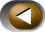## What is a tsunami?

Tsunami is a Japanese word meaning port wave.Fig. 1: How a seaquake wave behaves. As the depth of the sea decreases, so the speed and length of the wave decrease, while the height of the wave increases. (Credit: UNESCO - International Tsunami Information Centre)

Tsunamis can be defined as a series of waves of long wavelength originating from a vertical movement of the sea floor with the consequent displacement of a water mass. In deep ocean, waves travel at a speed of about 800 km/h and are only a few tens of centimetres high. The wavelength is a factor which distinguishes tsunamis from wind waves: a tsunami wavelength is considerably longer than a wind wave wavelength; it can be more than 200 km long. The wavelength is closely-linked to the sea depth. As the sea depth decreases, the wavelength decreases. At the same time, the height of the wave increases. Near the shore line the wave can assume the shape of a wall, up to tens of metres high, with a massive destructive power.

The speed of a tsunami wave can be simply expressed by the formulawhere g is the acceleration of gravity (9,8 m/s2), and h is the depth of the sea expressed in metres.

For example at an ocean depth of 4000 m the waves travel at about 700 km/h, i.e. the speed of a plane.

Fig. 1 shows how the speed and the wavelength of a tsunami wave change as soon as it reaches the coast. The height of the wave reaches its maximum on the shoreline.